tech@7universum.com
+7 (499) 938-98-57

# Experimental determination of the law of movement of introduction and exit links for liquid mixing

Экспериментальное определение закона движения вводной и выходной тяги при смешивании жидкости
Цитировать:
Toshov B., Khamroev S. Experimental determination of the law of movement of introduction and exit links for liquid mixing // Universum: технические науки : электрон. научн. журн. 2021. 4(85). URL: https://7universum.com/ru/tech/archive/item/11530 (дата обращения: 08.05.2021).
Прочитать статью:

DOI: 10.32743/UniTech.2021.85.4-5.46-52

ABSTRACT

This article presents a block diagram of a mechanism for a liquid mixing device "Hinged coupling" and its model, showing the serviceability of the mechanism, the ability of its links to move, the law of motion of its input and output links was determined experimentally. The regression expression with the correlation coefficient corr = 0.99, which represents the found laws of motion, and the angular velocity of the input and output links, the ratio of the motion transmission were calculated using the Mathcad program.

АННОТАЦИЯ

В данной статье представлена ​​блок-схема механизма перемешивания жидкости «Шарнирная муфта» и его модель, показывающая исправность механизма, способность его звеньев двигаться, экспериментально определен закон движения его входных и выходных звеньев. Выражение регрессии с коэффициентом корреляции corr = 0,99, представляющим найденные законы движения, и угловой скоростью входного и выходного звеньев, соотношением передачи движения были рассчитаны с помощью программы Mathcad.

Keywords: hinged coupling, structure diagram, mechanism model, input and output links, law of motion, regression, angular velocity, motion transmission ratio.

Ключевые слова: шарнирная муфта, структурная схема, модель механизма, входные и выходные звенья, закон движения, регрессия, угловая скорость, коэффициент передачи движения.

Mixing accelerates the processes associated with heat and mass transfer. One of the most effective methods of intensification (acceleration, intensification) of technological processes in various fields of production, used in the practice of modern technologies, mixing in liquid and common processes (heating and cooling, melting, crystallization, extraction, absorption, electrolysis, chemical heterogeneous reactions) is one. It is also used in the production of food and medicinal products, in the preparation of medicines of the required composition used in agriculture, in the preparation of fodder, in various processes related to the purification of various products and water in the field of chemistry and microbiology, in metallurgy in the processing of rocks for the extraction of precious metals from mining, in the manufacture of modern non-metallic materials to replace steel and castings, in the production of substances used in the defense industry, in steels obtained in continuous casting machines, the mixing process is applied in cast steel and others to prevent defects in the nature of crystallization, compression and separation.

At the 15th European Mixing Conference on Mixing in St. Petersburg in 2015, it was noted that over the last 20 years, process experiments and computational methods have undergone tremendous changes. During this period, computational hydromechanics, one of the quantitative methods of visual determination in the study of the flow structure in devices that mix energy with a liquid, emerged as the main tool of modern research. In 2018, 97 articles and monographs on the theory and practice of mixing were reviewed and commented by V.M Barabash, R.M Abiyev and N.N Kulov. It states that various methods of mixing (mechanical, pneumatic, structuring, vibrating, magnetic, etc.) were used to intensify the process . The analysis shows that the most common of these methods is mechanical agitation, which is carried out using a rotating torch in the working area (fluid) of the main process apparatus, and its design is relatively simple (motor-reducer-electric motor, base, cuff, shaft and torch), energy and it differs from other methods by its cost-effectiveness, ease of use and maintenance, and accounts for 95% of those available in terms of application.

To date, the mixing devices used in production do not always fully meet the technological parameters required by industrial enterprises. The reasons for this are:

1. The complexity of the mixing process;
2. Difficulty of physical analysis of the mechanism of formation of processes and events in the working area of ​​the mixing device;
3. Lack of reliable measurement techniques to determine movement parameters in the process

This leads to large errors in the design and manufacture of mixing devices used in industry. Typically, most of the available mixers operate in a stationary mode  i.e. the working joint of the mechanism consists of a blade that rotates at a constant angular velocity. The blade in turn affects the medium (liquid + solid particle) to be mixed by rotating.

As a result of this effect, the liquid moves, the intensity of mixing is high around the working joint of the mixer and decreases as it moves away from it, but there are areas of the container where the liquid velocity is too small or zero, otherwise the mixing process does not take place. The degree of homogeneity of the prepared mixture is lost, the preparation time of the mixture at the desired concentration is prolonged, a lot of energy is expended. In addition, the speed of the mixing medium is the same as the speed of the working joint after a certain time, the relative motion between them disappears, resulting in the fluid moving in a rhythmic manner, separating the particles that make up the mixture (as in the case of a driver on a long road) sedimentation is observed.

The above is one of the main problems of the mixing process today. If a variable rotational motion is applied to the mixer, the mixing process is accelerated, the particles are in turbulent motion, accelerating or slowing down relative to the motion of the liquid in an instant. To put it more simply, if the fluid to be mixed has a changing kinematic parameter, i.e. a complex motion, the problems will start to solve on their own. This process can be done in different ways. In recent times, to intensify the mixing process, working bodies and mixing vessels have begun to use devices that generate complex, variable angular velocity, uneven complex movements . To create such a movement, it was first used in production by scientists at Kazan State Agrarian University as a result of research on the Bennett space mechanism model or devices based on it .

However, the constructions of the existing mechanisms have a complex structure and are driven by several drives, which have spherical hinges, so their service life is short. Furthermore, due to the lack of research on the mixing process, the spatial groups of the created devices have not been studied and the theory of mixer creation has not been developed due to the lack of a classification theory. One of the difficulties of using space mechanisms as mixers is the complexity of modeling them, applying them to production. As an example, 250 years ago, the Egyptian scientist Philo, in his book Mechanics, theoretically developed a spherical spatial four-joint mechanism in which the axes of the rotating hinges intersect at one point. After 1800, the Italian mathematician, philosopher and physician Giorolama Cardano created this mechanism and used it to set up marine compasses (applied to the manufacture of the mechanism he created today). Today, this spherical hinged mechanism is successfully used as an extension in the design of all cars. As another example, a four-joint spatial mechanism in which the rotating hinge is only cylindrical, the axes do not intersect, and are not parallel was theoretically developed in 1903 by the English mathematician Bennett, but he himself did not believe it would work because he could not create a model. This article has interested many foreign and local scientists, as similar five- and six-link spatial mechanisms have been developed in terms of geometry, but no model has been created. Only after 75 years, professors of the Kazan school B.V Shitikov and P.G Mudrov were able to create a model and production model of this spatial mechanism [2, 3]. This suggests that the application of spatial mechanisms to production is much more complex.

Our goal is for the mechanism to be created to act on the fluid in the horizontal and parallel planes, as well as in the vertical planes, just like the existing mechanical meshes. One of the ways to achieve our goal is the mechanism called "Hinged Coupling" , which we propose. The structural scheme of the mechanism is shown in Figure 1.a.

The device consists of a leading and a follower half-clutch, which are connected to each other by telescopic rods in the form of piston-cylinders, the axes of which intersect. Each half coupling consists of a fork and hook connected in the form of a hinge, and each connecting rod is connected in the form of a hinge with a hook of the first half coupling with one end and a hook of the other half coupling with the other end. Holes 10 are drilled in the outer parts of the connecting rods.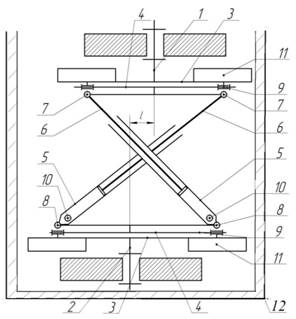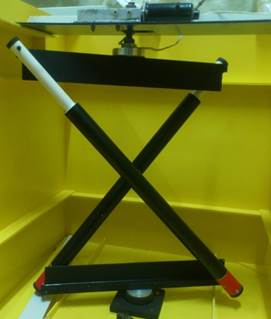а)                                                                                        б)

Figure 1. Block diagram (a) and model (b) of the hinged coupling

1, 2 - leading and following half couplings; 3-fork; 4 rings; 5, 6 - outer and inner (cylinder and piston) parts of the telescopic connecting rod; 7, 8 - cylindrical hinges, 9 hinge axis, 10 - holes drilled in the cylinder, 11 - mesh blades, 12 - cylindrical vessel filled with liquid to be mixed

The telescopic connecting rod, consisting of 5 outer and 6 inner parts, acts like a piston-cylinder and transmits rotational motion from the leading half-clutch to the following half-clutch.  The leading half-clutch has a l-length radial displacement (excess) relative to the driven half-clutch. The plug 3 and the ring 4 are connected to each other, the axis of which is connected by a hinge 9 perpendicular to the axis of rotation of the fork. The outer 5 and inner 6 parts of the telescopic connecting rod correspond to the ring 4, the axes are parallel to each other and the fork 3 and the hinge 9 are mounted perpendicular to the axis. A hole 10 is installed near the corresponding hinges of the telescopic connecting rod path (cylinder) 5 which are connected to the ring. This hinged coupling is mounted in a cylindrical vessel filled with the liquid to be mixed. During the operation of the coupling, there is a rotational motion in the leading half-coupling, the outer 5 and inner 6 parts are transmitted to the driven half-coupling 2 by means of two telescopic rods whose axes intersect at an angle to each other. The driven half-coupling rotates the liquids in the horizontal and parallel planes, mixing them in these planes. The telescopic connecting rod, on the other hand, moves back and forth along the outer 5 parts involved in the complex motion in the form of a cylinder, sucking and blowing the liquid through the hole 10 and mixing it in a vertical plane. As a result, the liquid mixes in both horizontal and vertical planes. This causes the liquid to be mixed to be sediment-free and a mixture of the same concentration to be formed. This mechanism, unlike other mechanisms, has two output links that move the fluid, the first of which is a driven half-clutch, and the second is an internal joint capable of absorbing and blowing liquid - a telescopic connecting rod and its path.

An analysis of the scientific and educational literature on machines and mechanisms has shown that many literatures and monographs are devoted to the single-motion mechanisms that are external to the input joint and the generalized force that drives it. Modern machine building is set to create a multifunctional machine that provides high reliability, accuracy and productivity. In mechanisms where the inlet steam is internal, the generated force is applied to its moving joint and naturally significantly increases the parameters required by the machine. These mechanisms are now used in hoisting and hauling, digging-driving, drilling, piling, road transport and construction machinery, as well as industrial robots and medicine .

A mechanism called the proposed hinged coupling  is spatial, and a model of it has been created to study its kinematics, to know how efficiently it works (Figure 1.b). When studying the structure and kinematics of spatial mechanisms , it was shown that the equation found for the angular velocity and angular acceleration of a connecting rod is universal and can be applied to a connecting rod of different structural parameters for the primary mechanism. Visual examination of the newly created mechanism or its strength, mobility, mobility and kinematics of the joints that make it up are often studied in the model of the mechanism [8, 9, 10]. Kinematic analysis of the mechanism, its input and output half-couplings, determination of the laws of motion of the telescopic connecting rod, is part of a set of parameters that indicate what it is capable of. There are many ways to determine these parameters, the most common being graphical, graph analytic, analytical, and experimental methods. The first three methods use a kinematic scheme of the mechanism. We used an experimental research method. From a methodological point of view, the experiment ensures the transition of research from slow activity to active activity. Experiments can be natural or artificial. Natural experiments are conducted to study social phenomena in production, life, and so on. Artificial experiments are widely used in engineering and other sciences. Depending on the nature of the object or process model under study, the selection and conduct of experiments, they are divided into laboratory and production type. Laboratory experiments are carried out on special modeling devices and stands using standard instruments and appropriate apparatus. In the experiment, it is possible to change the conditions of study of the object, to perform it in its pure form, to return it, and to study it in simplified, scaled-down models.

With the above in mind, a model of the mechanism shown in Figure 1.b was created and experimented with. In the mechanism, the radial displacement (excess) between the axes of the leading and driven semi-couplings is prepared by varying the length ((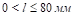).This means that as the distance l between the axes of the couplings increases, the path of the telescopic connecting rod also increases. As a result, there is a difference between the angular velocities of the input and output joints. Knowing which law this difference varies with is also one of the indicators that determine the ability of a mechanism.

The number of revolutions of the motor connected to the inlet clutch is n = 14 rpm. The half-couplings, which are the inlet and outlet joints, rotate in a horizontal plane. To measure their laws of motion, the degree to which the outlet joint is rotated in degrees and the distance traveled by the telescopic connecting rod when the inlet joint is rotated at an angle of about 100  we measured mm. Measurements were repeated 3 times. To do this, we fastened 360 ° conveyors with an accuracy of 10 to the walls where the axes of the inlet and outlet joints are mounted. The results were recorded in a notebook. Knowing the time taken for the input joint to rotate once, we calculated the angle of rotation of the joints by relating them to time. During the experiment, the radial displacement (extrusion) between the leading half-coupling and the leading half-coupling axes was maintained at l = 20 mm in length. The time variation of the angle of rotation of the input and output joints is shown in Figures 2 and 3, where φ1 and φ2 are the angles of rotation of the input and output joints, respectively, in degrees; t is the time taken for the joints to turn at an angle, sec. This article presents the results of experiments on input and output joints.

Graphical representation of the results of the experiment, the definition of its mathematical expression allows to better understand the physical nature of the process under study. This requires mathematical calculations. Today, modern computer mathematics, computer technology has become an integral part of all industries and professions, that is, has provided a whole package of integrated software systems [11, 12, 13] for the automation of mathematical calculations.  Among these systems, Mathcad [11, 12] is a simple, sufficiently elaborated and verified system of mathematical calculations. It includes the experiences, rules, and methods of mathematical calculation accumulated as a result of the development of mathematics over many years. We therefore used the Mathcad package to graphically represent the experimental results and to determine the algebraic expression of the functional dependence of the input and output joints on time.

The Mathcad package is a software tool for performing engineering calculations, which allows you to solve algebraic and differential equations with variable and invariant parameters, analyze functions, search for their extremums, build tables and graphs to analyze the solutions found. Simultaneously with the appearance of a discrete variable in Mathcad, it was possible to find the values ​​of functions for the values ​​of the variable and build tables and graphs without using programming operators based on the results obtained. Discrete variables are loop operators. Such variables can take sequential numbers that increase or decrease with a certain step. The results of the experiment (arrays) consist of one or more discrete variables and their function. In Mathcad, we enter the column and row numbers from the Matrix panel dialog box used to create the array, take the array template, and fill each space in it with discrete values ​​of the experimental results. It takes the form of a matrix in the form of a row. Now, to display them graphically, press the x-y Plot button on the Graph panel in the package and name the time t on the abscissa axis of the two-dimensional graphic template and the angle of rotation of the input and output joints φ11(t)and φ22(t)on the ordinate axis  names are placed. As a result, these connections are graphically represented in Figures 2 and 3 by colored circles and triangles, respectively.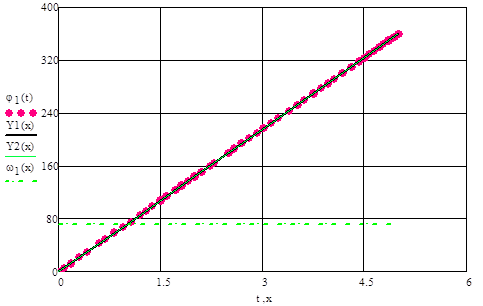Figure 2. The law of change of the angle of rotation of the inlet joint with time when the excess in the liquid mixer is l = 20 mm. Where: φ1(t) – `is the experimental result; Y1 (x), Y2 (x) are regression lines approximating the experimental data; ω1 is the angular velocity of this joint

As can be seen from Figures 2 and 3, the angle of rotation of the inlet and outlet joints increases in a straight line during one cycle. Typically, the purpose of conducting various experiments is to describe the experimental data in the form of a function, so that the arrays derived from it can be used in subsequent calculations. If the line we choose passes through all the experimental points, interpolation, if not, then the function that connects the experimental intermediate points is called regression. The meaning of regression is to determine the appearance of a function that approximates experimental data and to find the coefficients of this or that analytical relationship selected. The Mathcad program has two types of functions, several of which are set when selecting a regression function.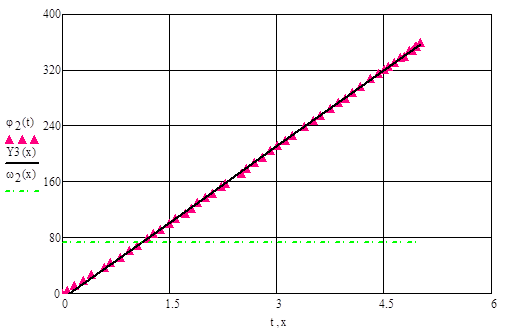Figure 3. The law of change of the angle of rotation of the outlet joint with time when the excess in the liquid mixer l = 20 mm. Where: φ2(t) – experimental results; Y3 (x)) - regression lines approximating experimental data; ω2 is the angular velocity of this joint.

From the representation of the experimental data in the graph, we selected their regression in the form of a function Y(x)=C0+C1x  given that the law of motion of the input and output joints of the mechanism is close to a straight line. Y1 (x) for the law of motion of the input joint using the line (x, y) and medfit (x, y) formulas of the straight line Y(x)=C0+C1x which minimizes the sum of the square error, which does not require the initial approximation of the experimental data The calculation of the coefficients C0 and C1 of the regression functions Y2 (x) and for the output link Y3 (x) in Mathcad is shown in Figure 4. Figure 4 also shows the correlation coefficients corr to estimate the relationship between the set of experimental data and the values ​​of the function that approximates them.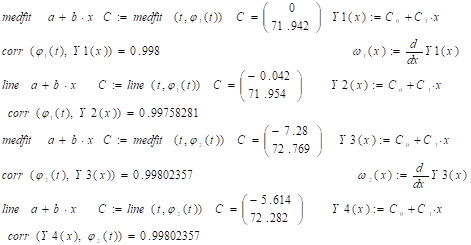Figure 4. Calculation of regression functions and correlation coefficients approximating experimental results in Mathcad

The regression lines approximating the experimental results from Figure 4 are calculated by the expression Y1 (x) = 71.972x, Y2 (x) = - 0.042 + 71.954x. which is shown in Figure 3. As can be seen from the graph, the experimental results and the regression lines overlapped. This is confirmed by the fact that the high correlation value (corr = 0.99) calculated in Figure 4 is very well represented by the straight-line equation in which the experimental data are selected and the coefficients are determined. So, using the regression functions, we would not make a big mistake if we wrote the laws of motion of the hinge coupling as input φ1 and output φ2 as follows: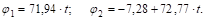By differentiating these laws once, we obtain their angular velocities.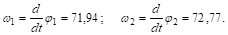The number of transmission ratios of the hinged coupling. This size is one of the main characteristics of the mechanism. If the generalized coordinate φ which determines the desired position of the joints of the mechanism, is known, the function i(φ) represents the interconnection of its input and output joints [14, 15]. In the design of the mechanism, it is known what the movement parameters of the output joint are. The motion parameters of the input link of the designed mechanism are determined by the transmission ratio.

Thus, based on the structural scheme of the "Hinged Coupling" designed for mixing liquids, its model was developed and its ability to move was confirmed. This mechanism, unlike other mechanisms, has one input and two output links. In order to apply it in production, the kinematics and dynamics of the model must be studied and design work must be done. This article examines the kinematics of the first of the input and output links of a model whose excess is constant. The next study will be devoted to the study of the law of motion of the second output link and to determine what changes these laws achieve as a result of the effect of the excess and their dynamics.

References:

1. Barabash V.M., Abiev R.Sh., Kulov N.N. Review of works on the theory and practice of mixing // Theoretical foundations of chemical technology. 2018.Vol. 52. No. 4. P. 367-383.
2. Mudrov A.G. Designs and model of mixing in apparatus with a stirrer // Izvestiya KGASU. 2018. No. 1 (43). S. 226-233.
3. Mudrov A.G., Mardanov R.Sh. Review of research on spatial mechanisms with rotary hinges // Theory of mechanisms and machines. 2015. Volume 13. No. 2 (26). S. 62-75.
4. Patent № US FAP 00861. Hinged coupling // Toshov B.R., Kushimov F., Toshov J.B., Eshboeva Z.N. 2013 y. Byul. Published in № 12.
5. Semenov Yu.A. Application of machines and mechanisms with internal inputs // Theory of mechanisms and machines. 2003. No. 1. S. 30-49.
6. Yarullin M.G., Mingazov M.R. Structural synthesis of a two-movable spatial 5R mechanism and elements of tracking control // Bulletin of the Samara Scientific Center of the Russian Academy of Sciences. 2014.Vol. 16.No. 6. P. 214-220.
7. Yarullin M.G., Mingazov M.R. Kinematics of characteristic points of the working links of the spatial 4R-mechanism as an activator of mixing processes // Bulletin of ISTU. 2014. No. 3 (63). S. 34-38.
8. Evgrafov A.N., Petrov G.N. Geometry and kinematics of the turbulent mixer mechanism // Modern mechanical engineering. Science and education. 2013.S. 701-708.
9. Mudrov A.G. Investigation of spatial mechanisms with a special structure // Bulletin of Kazan State Agrarian University. 2019. No. 2 (53). S. 111-116.
10. Semenov Yu.A., Semenova M.S. Features of the kinematic analysis of mechanisms with internal inputs // Theory of mechanisms and machines. 2004. Volume 2. No. 2. S. 30-39.
11. Makarov E. Engineering calculations in Mathcad 15: Training course. - SPb .: Peter. 2011 .-- 400 p.
12. Matsyuk I.N., Tretyakov V.M., Shlyakhov E.M. Analytical kinematics of flat lever mechanisms of high classes using Mathcad // Theory of mechanisms and machines. 2012. Volume 10. No. 1. P. 65-70.
13. Ioffe M.L. On the choice of the parameters of the Bennett mechanism and its modeling in MATLAB // Bulletin of higher educational institutions. Mechanical engineering. 2016. No. 2 (671). S. 11-19.
14. Toshov B.R., Khamroev Sh.G. Stirring of liquids with a changing diameter of a mechanical stirrer // Collection of scientific works of BNTU. Minsk. 2019.Vol. 2.S. 197-203.
15. Toshov B.R., Khamzaev A.A., Khamroev Sh.G. Kinematics of the hinge coupling used in a mechanical mixer // Young Scientist. 2017. No. 1 (135). S. 89-95.
Информация об авторахCandidate of physical and mathematical sciences, Navoi State Mining Institute, Uzbekistan, Navoi

канд. физ.-мат. наук, Навоийский государственный горный институт, Республика Узбекистан, г.НавоиAssistant, Navoi State Mining Institute, Uzbekistan, Navoi

ассистент, Нваоийский государственный горный институт, Республика Узбекистан, г.Навои

Журнал зарегистрирован Федеральной службой по надзору в сфере связи, информационных технологий и массовых коммуникаций (Роскомнадзор), регистрационный номер ЭЛ №ФС77-54434 от 17.06.2013
Учредитель журнала - ООО «МЦНО»
Главный редактор - Ахметов Сайранбек Махсутович.
Top